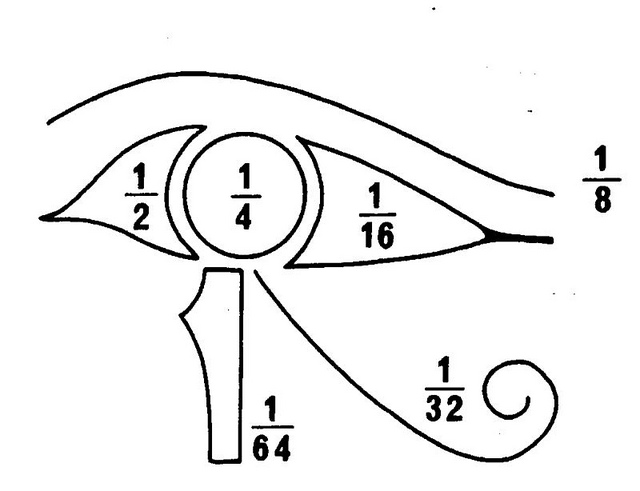Ancient Egyptians used only unit fractions, that is fractions with 1 as the numerator. They wrote all fractions as the sum of unit fractions with different denominators. We are going to explore how we can write the fractions we use in the form of Egyptian fractions. We shall use a method called the Greedy Algorithm invented by Fibonacci.

For example $$\frac{2}{5}=\frac{1}{4}+\frac{1}{10}+\frac{1}{20}$$.

Can you write $$\frac{5}{9}$$ as the sum of unit fractions?

That is not very difficult but what did the Egyptians do with fractions that we would write with large numerators such as $$\frac{61}{66}$$?

Using the Greedy Algorithm, so called because at each step you use the largest possible unit fraction that is smaller than the one you are working with, we see that the largest possible fraction smaller than $$\frac{61}{66}$$ is $$\frac{1}{2}$$ so the first step is $$\frac{61}{66}-\frac{1}{2}=\frac{28}{66}=\frac{14}{33}$$.

Then we subtract $$\frac{1}{3}$$ and get $$\frac{14}{33}-\frac{1}{3}=\frac{3}{33}= \frac{1}{11}$$ so $$\frac{61}{66}=\frac{1}{2}+\frac{1}{3}+\frac{1}{11}$$.

Now if you try this method on $$\frac{7}{15}$$ you should get $$\frac{7}{15}=\frac{1}{3}+\frac{1}{8}+\frac{1}{120}$$. Try it.

Next write $$\frac{61}{84}$$ as the sum of unit fractions.

Now choose some fractions of your own and see if you can make them into sums of unit fractions.

Does the greedy algorithm always work? Can all fractions be expressed as a sum of different unit fractions by applying the Greedy Algorithm?
Can you explain why?

Tagged with: